>
>
>
>
海斯迪克HKW-225
自营
客服关注微店

手机下单

•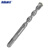•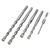•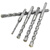•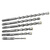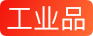海斯迪克 HKW-225 加长电锤钻头 冲击钻头 穿墙混凝土合金钻头 方柄四坑 18*500mm（1个）
京 东 价
降价通知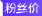批量购更低价

累计评价

0
增值业务
配 送 至
--请选择--
支持
选择规格
本地活动

# 其他型号

• 50个型号
• |
• 如需查看具体型号的属性差异，请点击商品名称进行查阅。
• 商品
规格
• 电锤钻头
• 电锤钻头
• 电锤钻头
• 电锤钻头
• 电锤钻头
• 电锤钻头
• 电锤钻头
• 电锤钻头
• 电锤钻头
• 电锤钻头
• 电锤钻头
• 电锤钻头
• 电锤钻头
• 电锤钻头
• 电锤钻头
• 电锤钻头
• 电锤钻头
• 电锤钻头
• 电锤钻头
• 电锤钻头
• 电锤钻头
• 电锤钻头
• 电锤钻头
• 电锤钻头
• 电锤钻头
• 电锤钻头
• 电锤钻头
• 电锤钻头
• 电锤钻头
• 电锤钻头
• 电锤钻头
• 电锤钻头
• 电锤钻头
• 电锤钻头
• 电锤钻头
• 电锤钻头
• 电锤钻头
• 电锤钻头
• 电锤钻头
• 电锤钻头
• 电锤钻头
• 电锤钻头
• 电锤钻头
• 电锤钻头
• 电锤钻头
• 电锤钻头
• 电锤钻头
• 电锤钻头
• 电锤钻头
• 电锤钻头
价格
库存
选购数量
• 电锤钻头
- +
• 电锤钻头
- +
• 电锤钻头
- +
• 电锤钻头
- +
• 电锤钻头
- +
• 电锤钻头
- +
• 电锤钻头
- +
• 电锤钻头
- +
• 电锤钻头
- +
• 电锤钻头
- +
• 电锤钻头
- +
• 电锤钻头
- +
• 电锤钻头
- +
• 电锤钻头
- +
• 电锤钻头
- +
• 电锤钻头
- +
• 电锤钻头
- +
• 电锤钻头
- +
• 电锤钻头
- +
• 电锤钻头
- +
• 电锤钻头
- +
• 电锤钻头
- +
• 电锤钻头
- +
• 电锤钻头
- +
• 电锤钻头
- +
• 电锤钻头
- +
• 电锤钻头
- +
• 电锤钻头
- +
• 电锤钻头
- +
• 电锤钻头
- +
• 电锤钻头
- +
• 电锤钻头
- +
• 电锤钻头
- +
• 电锤钻头
- +
• 电锤钻头
- +
• 电锤钻头
- +
• 电锤钻头
- +
• 电锤钻头
- +
• 电锤钻头
- +
• 电锤钻头
- +
• 电锤钻头
- +
• 电锤钻头
- +
• 电锤钻头
- +
• 电锤钻头
- +
• 电锤钻头
- +
• 电锤钻头
- +
• 电锤钻头
- +
• 电锤钻头
- +
• 电锤钻头
- +
• 电锤钻头
- +
• 请先选择商品数量
• 0
• |
• 0.00

### 为你推荐

• 店长推荐
• 店铺热销
• 热门关注
• 商品介绍
• 规格与包装
• 售后保障
• 商品评价
• 本店好评商品海斯迪克 HKW-225 加长电锤钻头 冲击钻头 穿墙混凝土合金钻头 方柄四坑 18*500mm（1个）
X
• 商品名称：海斯迪克HKW-225
• 商品编号：100011080914
• 商品毛重：1.0kg
• 商品产地：中国大陆
• 刀柄/刀柄附件：其他
• 铣削刀具：其他
• 孔加工刀具：其他
• 切断刀具：其他
• 车削刀具：其他
• 机夹焊接刀片：其他
• 螺纹加工刀具：其他